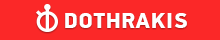GMAT Question of the Day - Daily to your Mailbox; hard ones only

 It is currently 26 Jun 2019, 11:23GMAT Club Daily Prep

Thank you for using the timer - this advanced tool can estimate your performance and suggest more practice questions. We have subscribed you to Daily Prep Questions via email.

Customized
for You

we will pick new questions that match your level based on your Timer History

Track

every week, we’ll send you an estimated GMAT score based on your performance

Practice
Pays

we will pick new questions that match your level based on your Timer History

Not interested in getting valuable practice questions and articles delivered to your email? No problem, unsubscribe here.A shop sells cigars of brand A as well as brand B. Let X be the event

Author Message
TAGS:

Hide Tags

Retired ModeratorP
Joined: 22 Aug 2013
Posts: 1437
Location: India
A shop sells cigars of brand A as well as brand B. Let X be the event  [#permalink]

Show Tags

400:00

Difficulty:95% (hard)

Question Stats:33% (02:12) correct67% (01:54) wrongbased on 60 sessions

HideShow timer StatisticsA shop sells cigars of brand A as well as brand B, among other brands. Let X be the event that a customer buys at least one cigar of brand A, let Y be the event that a customer buys at least one cigar of brand B, and let Z be the event that a customer buys cigar of neither brand A nor brand B. Probability of event X is denoted as P(X), probability of event Y is denoted as P(Y), probability of event Z is denoted as P(Z). What is the probability of X U Y, P(XUY) i.e., what is the probability of either event X or event Y.

(1) P(Z) = 0.35.

(2) P(X) = 0.40 and P(Y) = 0.38.
CEOP
Joined: 18 Aug 2017
Posts: 3947
Location: India
Concentration: Sustainability, Marketing
GPA: 4
WE: Marketing (Energy and Utilities)
A shop sells cigars of brand A as well as brand B. Let X be the event  [#permalink]

Show Tags

1
#1:

p(XuY) = p(X) + p(Y) - p(XnY)
or
p(XuY) = 1 - p(XnY)' = 1 - Probability of neither of the events occurring = 1 - p(Z)
P(Z) = 0.35 - Sufficient

#2:
P(x)=P(a)=0.4 and P (y)=P(b)=0.38
P(a)+P(b)= 0.4+.38 = 0.78; not sufficient

IMO A

amanvermagmat wrote:
A shop sells cigars of brand A as well as brand B, among other brands. Let X be the event that a customer buys at least one cigar of brand A, let Y be the event that a customer buys at least one cigar of brand B, and let Z be the event that a customer buys cigar of neither brand A nor brand B. Probability of event X is denoted as P(X), probability of event Y is denoted as P(Y), probability of event Z is denoted as P(Z). What is the probability of X U Y, P(XUY) i.e., what is the probability of either event X or event Y.

(1) P(Z) = 0.35.

(2) P(X) = 0.40 and P(Y) = 0.38.

_________________
If you liked my solution then please give Kudos. Kudos encourage active discussions.

Originally posted by Archit3110 on 09 Jan 2019, 06:28.
Last edited by Archit3110 on 10 Jan 2019, 08:48, edited 1 time in total.
ManagerG
Joined: 02 Aug 2015
Posts: 153
Re: A shop sells cigars of brand A as well as brand B. Let X be the event  [#permalink]

Show Tags

amanvermagmat wrote:
A shop sells cigars of brand A as well as brand B, among other brands. Let X be the event that a customer buys at least one cigar of brand A, let Y be the event that a customer buys at least one cigar of brand B, and let Z be the event that a customer buys cigar of neither brand A nor brand B. Probability of event X is denoted as P(X), probability of event Y is denoted as P(Y), probability of event Z is denoted as P(Z). What is the probability of X U Y, P(XUY) i.e., what is the probability of either event X or event Y.

(1) P(Z) = 0.35.

(2) P(X) = 0.40 and P(Y) = 0.38.

p(XuY) = p(X) + p(Y) - p(XnY)
or
p(XuY) = 1 - p(XnY)' = 1 - Probability of neither of the events occurring = 1 - p(Z)

(1) P(Z) = 0.35 - Sufficient

(2) P(X) = 0.40 and P(Y) = 0.38 - We don't know p(XnY) - Not sufficient.

Hence I'll go with A.

Cheers!Re: A shop sells cigars of brand A as well as brand B. Let X be the event   [#permalink] 09 Jan 2019, 11:31
Display posts from previous: Sort by

A shop sells cigars of brand A as well as brand B. Let X be the event